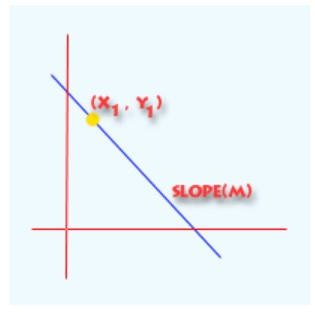# Point Slope Form Calculator

Enter the values of X1, Y1, and (m) in the below input boxes and hit the Calculate button to get the equation of a straight line using point slope form calculator.

Formula:
( y - y1 ) = m ( x - x1 )

Give Us Feedback

## Point Slope form Calculator

Point slope calculator is an online tool developed to find the equation of a straight line using the slope and point on that line or two points.

## What is point-slope?

Point slope form is a general form for linear equations. The equation of a line can be determined by using the point-slope form or slope intercept form. The point slope form highlights the slope of the line and a point on the line.

The slope of the line is the ratio of the change in the values of the y-axis and the change in the values of the x-axis [`(y2 – y1)/(x2 – x1)`]. It is used to evaluate the steepness of the line.

You can find the equation of point-slope form in the below section.## Point slope formula

The point slope equation can be expressed as:

`y - y1 = m(x- x1)`

Where,

• `m` is the slope, and
• `x1, y1` are the coordinates of a point.

## How to find the equation of a line?

To find the equation of a straight line without a point slope form calculator, follow the below examples.

Example 1: For 1 point & slope

Find the equation of a line whose known points are `(2, 3)` and the slope of line is 8.

Solution:

Step 1: Identify and write down the values.

x1 = 2

y1 = 3

`m = 8`

Step 2: Place the values in the point slope formula and solve the equation.

y - y1 = m(x- x1)

`y – 3 = 8(x - 2)`

Further simplification

y – 3 = 8x – 16

8x – 16 – y + 3 = 0

8x – 13 – y = 0

`y = 8x – 13`

Example 2: For 2 points

Evaluate the linear equation of the line whose coordinate points are `(10, 15)` and `(12, 20)`

Solution:

Step 1: Write the given points of the line.

x1 = 10

x2 = 12

y1 = 15

y2 = 20

Step 2: Evaluate the slope of the line.

m = (y2 – y1)/(x2 – x1)

m = (20 - 15)/(12 - 10)

`m = 5/2 = 2.5`

Step 3: Now place the slope and 1 point of the line to the point-slope equation.

y - y1 = m(x- x1)

`y - 15 = 2.5(x - 10)`

Further simplification

y - 15 = 2.5x - 25

y = 2.5x - 25 + 15

`y = 2.5x - 10`

## FAQs

### How to convert slope intercept form to point slope form?

Below are the steps to convert `y=mx+c` to `y−y1=m(x − x1)`:

• Determine the Slope and Y-intercept: From the equation `y=mx+c`, determine the values of slope “m” and y-intercept (c).
• Use the Y-intercept as the Point: In the slope-intercept form, you always have a known point on the line: the y-intercept `(0, c)`. You can use this point to write the equation in point-slope form.
• Input values into Point-Slope Formula: Use the y-intercept `(0, c)` as your `x1,y1`​ in the point-slope formula. `y−y1​=m(x−x1​)`

### How to find the equation of a line with slope and point?

To find the equation of a line given a slope “`m`” and a point `(x1, y1​)`, you can use the point-slope form of a linear equation:

Let's explore how to use it:

• Input the given values: Insert the known values for the slope “m” and the point (x1, y1) into the point-slope formula.
• Simplify the equation (optional): You can rearrange the equation to get it in slope-intercept form `y=mx+c` or standard form `Ax+By=C`, depending on your preference or the requirements of your task.

Example:

Given the point `(2, 3)` and a slope `m = 4`, let's find the equation of the line.

Solution

Input the values:

Using the point-slope form: y−3=4(x − 2)

simplify:

y−3=4x−8

y=4x−5

So, the equation of the line in slope-intercept form is `y = 4x − 5`.

### How to convert the point-slope form to the standard form Ax+By=C?

• Start with the point-slope form: `y − y1 ​= m(x − x1​)`.
• Distribute the right side: `y − y1 ​= mx − mx1`.
• Rearrange the terms to get the equation in the form `Ax + By = C `If “m” is a fraction, multiply every term by the denominator to eliminate fractions.

### How to graph the line from the point and the slope?

• Plot the given point `(x1, y1​) `on the graph.
• From this point, use the slope to determine a second point:
1. Rise = Numerator of the slope
2. Run = Denominator of the slope

For example, if the slope m = 2/3, rise 2 units up and run 3 units to the right to find your second point.

• Draw a straight line through the two points. Extend the line in both directions.

### How to convert the point-slope form to the slope-intercept form y=mx+b?

• Begin with the point-slope form: `y − y1​=m(x − x1​)`.
• Solve for y by rearranging the terms: `y = mx − mx1 ​+ y1`.
• The equation will now be in the form `y = mx + c`, where c is the y-intercept.